﻿ Developing Mathematics Problem Based on PISA Level of Space and Shape Content to Measure Student’s Mathematics Problem Solving AbilityPublications are Open
Access in this journal
Article Versions
Export Article
• Normal Style
• MLA Style
• APA Style
• Chicago Style
Research Article
Open Access Peer-reviewed

### Developing Mathematics Problem Based on PISA Level of Space and Shape Content to Measure Student’s Mathematics Problem Solving Ability

Riski Sahrida Nasution , Kms. Muhammad Amin Fauzi, Edi Syahputra
American Journal of Educational Research. 2019, 7(10), 660-669. DOI: 10.12691/education-7-10-1
Received August 18, 2019; Revised September 21, 2019; Accepted October 05, 2019

### Abstract

This research aims to produce mathematics problems based on the PISA level content of Space and Shape with valid and practical procedures for developing problems and measure students' mathematics Problem-solving ability. The method used in this research is the development research method. This research consists of two stages, namely preliminary and formative evaluation stages which include self-evaluation, expert reviews, one-to-one, small groups, and field tests. Data collection techniques are based on walk-throughs, documents, tests, and questionnaires. After going through the one-to-one, small group, and descriptive validation stages, the questions were tested in the field test stage in class VIII of SMP Negeri 1 Padangsidimpuan. The results show the average value of mathematics problem-solving ability is 56.07 and it is categorized as Enough mathematics problem-solving ability, although there are still students who categorized in low. From these results, it can be said that developed PISA level questions are categorized as valid and practical criteria and have a potential effect on students' mathematics problem-solving abilities.

### 1. Introduction

Mathematics is a universal science that underlies the development of modern technology, has an important role in various disciplines and develops human thought. The rapid information and communication technology today is based on the development of mathematics in number theory, algebra, analysis, probability theory, and discrete mathematics. However, in reality, Indonesia only has a fairly low mathematical ability. Many factors affect the low mathematical ability in Indonesia, namely teachers lack mastery in understanding the 2013 curriculum, students are less trained in working on problems with the characteristics of the Program for International Assessment (PISA), students are lack understanding in solving contextual problems. The 2013 curriculum requires students to be more independent and students can solve math problems related to daily life. The development of mathematics problems in this globalization era refers to the PISA problem. Indonesia in dealing with PISA mathematics problems is still experiencing difficulties. The involvement of PISA is to see the extent to which programs in Indonesia are developing compared to other countries. Also, exercises like PISA problems can improve students' reasoning ability.

PISA is an international standard study organized by OECD (Organization for Economic Cooperation and Development) which studies student’s mathematical literacy ability 1. According to 2, mathematical literacy is the ability of individuals to formulate, apply, and interpret mathematics in various contexts. It is including mathematical reasoning and using mathematical concepts, procedures, facts and mathematical tools to describe, explain and predict phenomena/ events. PISA provides important information about student skills at the junior high school level, which is about 15 years old students in using mathematics in daily life 3. PISA uses an innovative literacy approach, a learning concept that is related to students' ability to apply knowledge and skills in key subjects accompanied by the ability to study, reason, communicate effectively and solve and interpret problems in various situations 4. PISA is designed to find out whether students can use their mathematical potential in real life in society through a contextual mathematical learning concept 5. Factors that influence the successfulness of research with PISA level problems are: (a) Students have not been able to adjust PISA problems, because adjusting PISA questions requires much time and effort. This statement is supported by 6 which states that one of the factors causing the low mathematical literacy ability of Indonesian students is not being trained in solving problems with characteristics of PISA problems; (b) The ability of students to construct contextual problems into mathematical contexts is still considered to below. This is very influential because the PISA problem starts with a contextual and real problem. PISA does not only make students learn about mathematics in real life but also practice students' mathematical literacy ability according to their age. Therefore, one of the efforts that can be done is to encourage teachers and students to plan a PISA- based learning and design a learning evaluation which is by the characteristics of PISA 7. Besides, teachers are also required to be able to design the problem using a context which is close to the life of their students 8.

Various researches on the development of mathematical PISA-like problems for High School students with various focus both on content and ability have been conducted. For example, 9 Pengembangan Soal Matematika Model PISA Berbasis Online; 10 Pengembangan Soal Model PISA Untuk Mengetahui Profil Literasi Matematis Siswa SMA; 11 also wrote a journal entitled Pengembangan Soal Matematika Model PISA Untuk Program Pengayaan Kelas VII SMP; 12 developed the PISA problem to measure students' mathematical communication ability; 13 developed level 6 PISA problem for junior high school; 14 developed the PISA-like mathematics task with the context of Indonesian natural and cultural heritage. This shows that many parties are interested and consider that the development of problem-based on PISA level is very necessary. Based on the mathematical framework of PISA 2015, the mathematical content in PISA consists of changes and relationships, spaces and shapes, numbers, and uncertainties and data 15. Various contents are more specific in it, such as geometry, number patterns, algebra, probabilities and uncertainties, and data. Based on the survey Program for International Students Assessment (PISA) 2000/2001, it shows that students are weak in geometry, especially in understanding space and shape 16. Likewise, researchers note that students experience difficulties and show poor performance in learning geometry. Usiskin stated that many students failed to understand key concepts in geometry 17. Stated that many of the weaknesses in Indonesian students' mathematical abilities are revealed in the results of the PISA study 18. In general, students' weaknesses are not yet able to develop their reasoning ability, do not have the habit of reading while thinking and working in order to understand essential and strategic information in solving problems, and still tend to "accept" information and then forget it, so mathematics subjects have not been able to become a "school of thinking" for students. According to 19, the factors that cause students' mistakes in solving PISA problems are low students' reasoning and creativity in solving real context problems and manipulating them into mathematical form. Mathematical reasoning is one of the basic abilities needed in mathematical literacy 20.

Based on the results achieved by Indonesia in PISA, it shows that students in Indonesia are still ranked 63 out of 72 countries for mathematics literacy 21. One of the causes of the low ability of mathematics literacy is the lack of availability of learning tools that can support the improvement of mathematical literacy ability, including the use of learning instruments or student activity sheets that do not present assignments to improve their literacy ability 22. Given the importance of problem-solving, so the NCTM 23 states that problem-solving is a major focus in mathematics learning. Problem-solving skills are the ability that students learn from mathematics, so that practice is part of solving math problems. The more students are experienced in solving problems, the better the problem-solving skills it has. According to Mayer 24 problem solving as cognitive processing directed at transforming a given situation into a goal situation when no obvious method of Solution is available. Means resolution as cognitive processing is geared towards changing certain situations into a destination situation when no obvious solution method is available.

Model learning of mathematics at this time affected the more conventional view that the mathematical tools at its disposal. This view encourages teachers are inclined to say concepts/theorems and how to use them. Teachers tend to transfer knowledge to students and students' minds to accept passively and uncritically. This attitude often gives the deadlock think students where students can use the formula but do not know where it came from that formula and why the formula was used, in other words, students can only use the formula as a tool to answer questions and instead finding solutions and solving of problems that arise 25. According to 26 The purpose of problem-solving is given to students is: (a) It can elicit curiosity and motivation, cultivating the nature of creativity; (b) In addition to having the knowledge and skills (counting, etc.) is required the ability to skillfully read and make correct statements; (c) may give rise to genuine, new, distinctive, and varied answers, and may add new knowledge or can improve the application of science that has been acquired; (d) Inviting students to have problem-solving procedures, able to make analysts the troubleshooting indicators used by PISA 2003 27 are (1) Understand the Problem, (2) Characteristic the Problem, (3) Represent the Problem, (4) Solve the Problem, (5) Reflect on the Solution, (6) Communicate the Problem Solution. Thus, from these six indicators, researchers will limit the indicators to be used 1) Understand the Problem, 2) Represent the Problem, 3) Solve the Problem.

In 2012 PISA, Indonesia was only slightly better than Peru which was ranked lowest. The average mathematics score of Indonesian children was 375. Indonesia ranks 64 out of 65 countries with an average score of 375, while the average international score is 500 28. This shows the ability of Indonesian students to solve problems that demand the ability to study, give reasons, communicate effectively, and to solve and interpret problems in various situations is still low. Regarding 29, to help teachers to implement problems based on the PISA level is to provide students with bank problems of the PISA level. From the description above it can be concluded that problems based on PISA level do not only require the ability to apply concepts but rather how they can be applied in various situations and students' reasoning ability, solve problems and argue about how they can be solved. Based on some of the descriptions above, this research was conducted in SMP VIII to know and recognize students' mathematics problem-solving ability from the results of solving the problem based on PISA level on Space and Shape.

The results of this research are expected to provide information to the school about the ability of students in the discipline of mathematics so that it can help to improve student abilities and the quality of mathematics. This research was using a problem based on the PISA level on Space and Shape topic because it consists of the mathematics problem solving of students at the junior high school level. An interesting problem to be examined in this research is the Developing of Mathematics Problem Based on PISA Level of Space and Shape to Measure Students' Mathematics Problem Solving Ability in SMP Negeri 1 Padangsidimpuan.

### 2. Research Methodology

This research was conducted at SMP Negeri 1 Padangsidimpuan in class VIII in the second semester of the academic year 2018/2019. The type of research is a research & development (Design Research). The development model used is the Tessmer development model type of formative evaluation 30. In the formative evaluation stage following the developing flow, the steps included self-evaluation, expert reviews, one-to-one, small group, and field test 31. The preliminary stage is the preparation stage that the researcher will undertake to determine the place and subject of the research by contacting the principal and teacher to arrange a schedule and procedure for collaboration. The next stage is prototyping were the products that have been made or designed to be evaluated. The design results of the first prototype were developed based on self-evaluation which given to experts (Expert Review), students ( One-to-one ) and small groups in parallel. At this stage comments or suggestions as well as the results of trials on the first prototype serve as the basis for revising the design of the first prototype. The revised results tested on research subjects as a field test. Trials at this stage of the product were tried out to students of class VIII of SMP Negeri 1 Padangsidimpuan who were subjected to field trials. Furthermore, the data obtained from the field test was analyzed through reliability, difficulty level, and distinguishing features.

The data collection techniques used are walkthrough, documents, and tests of PISA problems on space and shape to measure students' problem-solving ability. Data analysis techniques used in the test instrument of mathematics problem-solving ability are the analysis of contents problems validity, analysis of students' questionnaires, reliability testing, difficulty level test, distinguishing power, and data analysis of mathematical reasoning ability.

Validity refers to the Measuring instrument against the mastery of the concept being measured so that it actually measures what should be measured. To calculate the validity of the test. 32 suggests a formula used to calculate validity, namely the correlation of product moments as follows: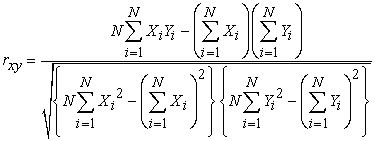Description: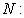Number of student taking the test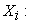Scores Earned on each item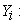Total scored of each item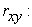Coefficient of validity test.

Next, to determine the validity of a test item, it is used to test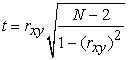with the criteria to be met is if thitung> ttabel with ttabel = t(1-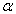)(dk) for dk = N-2 and α (significant level) is selected 5% The Instrument is valid, can the interpretation of the correlation coefficient used criteria according to 32 is as Table 1 follows:

To interpret the validity price of each item, the price is consulted on the Critique Price table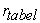product-moment with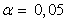. If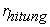>, The test is considered valid.

For the calculation of the test reliability, 33 suggests that the reliability coefficient of test form description (Essay test) can be used Alpha formula, as follows: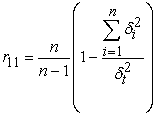Description: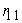: Overall test reliability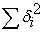: Number of score variances of each problem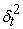: Total Variance

N: Number of items in the test.

The index of this difficulty level is generally expressed in proportion to the magnitude of 0.00-1.00. The larger the index of the difficulty level gained from the count result, the easier it is. A question of having TK = 0.00 means that no student is properly answered and if having TK = 1.00 means that the student is right. The formula below is used for description. The formula is like the following: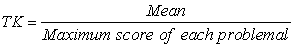With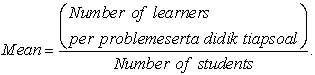The distinguishing Power Index of each item is usually also expressed in proportion. The differentiator Power Index ranges from-1.00 to + 1.00. The higher the differentiator's power, the stronger/better the problem. To know the differentiator about the description form is to use the following formula.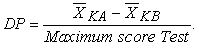The criteria are as follows:

The Data obtained from the results of the reasoning ability Test and the student response poll on the test are analyzed to know the skills of mathematics problem solving of the students, by comparing the average score students gained and the average score for each Mathematics problem-solving ability Indicators of the test results. Scores of mathematics problem-solving ability students are the number of scores students gained when completing mathematics problem-solving ability tests. The final value that the student acquired is: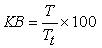Description:

KB: Learning Submission

T: Number of scores earned by students

Tt: Total Score Number

The capability Test Data is analyzed to determine the category of levels of students' mathematics problem-solving ability. The category of mathematics problem-solving ability of the student is determined as in the following table:

To determine the positive presence of students ' responses to the problem of PISA, student response data is analyzed in a quantitatively descriptive form of percentages and grouped for each indicator. The student response percentage is calculated using the formula 34.

Student response percentage =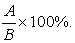Description:

Positive The proportion of students who respond positively

B: Number of students who are respondents.

Interpretation of the average poll score on Table 6 using the modified Likert scale of 35.

At least 80% of many of the subjects studied gave a positive response to the materials that had been developed.

### 3. Results and Discussion

This research is development research, so the product of this research is a learning tool in the form of problem-based on PISA level. The purpose of this development research is to describe: (1) the validity of developing mathematics problem based on PISA level in Shape and Space at class VII of SMP Negeri 1 Padangsidimpuan; (2) the practicality developing mathematics problem based on PISA level in Shape and Space at class VII of SMP Negeri 1 Padangsidimpuan; (3) procedure for developing mathematics problem based on PISA level in Shape and Space; (4) describe the measurement of students' problem solving ability at SMP Negeri 1 Padangsidimpuan through the problem based on PISA level in Shape and Space. Analysis of the data and research results obtained for each stage of the Tessmer development model are presented as follows.

3.1. Description of the Preliminary Stage

This stage begins with the collection of several references related to this research, namely research development, test instruments, and students' mathematics problem-solving ability. The next step is determining the place and subject of the research trial. The place of trial in this research was SMP Negeri 1 Padangsidimpuan. While the test subjects in this research were students of class VIII-3 of SMP Negeri 1 Padangsidimpuan. After determining the place and subject of the trial, observations were made aimed at identifying learning activities and students' mathematics problem-solving ability at SMP Negeri 1 Padangsidimpuan class VIII-3.

3.2. Description of Self Evaluation Stages

This stage has 4 activities, namely curriculum analysis, material analysis, student analysis, and design. Curriculum analysis was carried out to establish the basic problems needed in developing test instruments so that it can measure students' mathematics problem-solving abilities. The development of a problem based on PISA level in Space and Shape refers to the 2013 Curriculum. Student analysis focused on students of class VIII-3 as a test subject because the material was studied in class VIII. The average number of students in each class was 28 students. Based on observations and interviews with mathematics teachers, it can be seen that the mathematics knowledge of grade VIII-3 students was varied. There were less, medium and high abilities. This showed that there were different factors of interest for each student towards mathematics. In general, students' mathematics problem-solving ability has never been explored either by the teacher or other researchers. Material analysis is an activity to identify the main concepts that will be used in tests on grade VIII SMP.

Based on the curriculum analysis, it was found that the material to be used in the development of problem-based PISA levels in the Space and Shape which following the material in the 2013 curriculum was cubes and beams. After the material analysis activity is carried out the next step is to design problem based on PISA level in Space and Shape of high level thinking skills, including: test grids, test questions, answer sheets and assessment guidelines as consideration for the validator to check the validity of the content, construct and language of the mathematical problem solving ability test instrument. The test instrument grid is designed based on reasoning indicators for each question, the answer sheet is designed to contain steps for solving each problem to practice mathematical Problem Solving ability.

3.2.1. Description of Prototyping Phase (Validation, Evaluation, and Revision)

The purpose of this prototyping stage was to produce prototype II of the revised test instrument based on expert review and data obtained from one-to-one trials. Expert Review was used as a basis for revising and refining prototypes. The instrument validation was carried out by providing a validation sheet of test instruments, test questions, and answer sheets to the validator, which consisted of three mathematics lecturers from Universitas Negeri Medan and two mathematics teachers at SMP Negeri 1 Padangsidimpuan.

Suggested revisions from validator to the instrument which includes a test grid, test questions, test answer sheets, and student answer keys, can be seen in Table 7 below.

Comments and suggestions from the validator were used as consideration for revising the prototype of the test instrument to produce a second prototype.

3.2.2. One to one

In addition to the mathematical problem-solving ability test questions validated by experts, the test was also tested one to one on several students at SMP Negeri 1 Padangsidimpuan. These students were 3 non-subjects students who in high, medium, and low ability. The picture below is the answer of the first and second subjects with a high and medium ability on the answer sheet and can be seen that the answer is correct.

• Figure 1. Problem and Answer Number 1 of the Pisa Model for Space and Shape

From interviews with S1 and S2, they were able to explain that the number of points on the dice that appeared from below was 15 points. They were able to associate two-dimensional images with actual dice shapes (3 dimensions). This meant that both subjects were relating the problem to their real life. When interviewing, the researcher asked, "Bukankah yang Nampak pada Sisi Bawah Tidak Kelihatan Hanya Angka yang di Atas yang Kelihatan?" (Don't you see what appears on the underside are only the numbers above that appear). The answers to the two subjects were almost the same. They understood the shape of the dice in a real context.

The students' comments on completing the PISA questions in the one-to-one stage can be seen in Table 8 below.

Small-Group

In addition to the mathematical problem-solving ability test instrument, questions were validated by experts and tested on one to one the questions were also trialed by Small Group on several students of SMP Negeri 1 Padangsidimpuan. These students were 6 non-subject in high, medium, and low ability based on the learning outcomes known by a mathematics teacher.

The picture below is a question and answer sheet from one of the subjects in the small group.

Based on Figure 2 showed that student only wrote the formula without answering what the problem was meant. In the process of solving these problems, students were not careful enough so the answer was wrong.

• Figure 2. Problem and Answer Number 4 Pisa Model for Space and Shape

Field Test Stage

The prototype which has been validated and revised (prototype II), was tested on the subject of research trials, namely students of class VIII-3 of SMP Negeri 1 Padangsidimpuan. The class contained 23 students, but at the time of the trial, there was one student who did not attend school. Tes activities carried out for 2x40 minutes. The results of the test answers obtained from the work of students of class VIII-3 of SMP Negeri 1 Padangsidimpuan were analyzed to measure or determine the level of mathematical problem-solving ability of students. Besides, based on the results of student work will also be analyzed the reliability of the developed test instruments, in this stage also revised the instruments that have been tested to produce an appropriate product.

3.3. Analysis of Test Item Validation Test Results

The validity of the questions was analyzed by using Pearson's product-moment correlation formula by correlating the score of the item items with the total score. The results of testing the test instrument for understanding comprehension are presented in Table 10 below.

Table 10 is a research instrument testing of reasoning ability test for six essay questions with a significance level of 5%, dk = 24, obtained t table = 2.0555. If referring to the testing criteria, the test criteria are tcount > ttabel, then the mathematical problem-solving ability test can be used or valid. Thus based on calculations performed by manual, excel, and SPSS 22, it is concluded that the problem-solving ability test can be used or valid.

3.4. Results of Analysis of Student Responses

Based on the results of the questionnaire analysis of student responses one to one stage can be seen that the average results of all statements stated a positive answer of 66.7% while 33.3% of students stated a negative answer. While in the small group stage the positive answers were 66.7% and 21.2% stated negative answers. Student responses on field tests also showed more positive student responses than negative students' responses that were 72, 3% and 27.3%.

• Figure 3. Analysis of Students Response of One to One, Small Group and Fiel Test
3.5. Difficulty Level Analysis

The following results of the analysis of the level of difficulty items about PISA mathematical problem-solving ability can be seen in Table 11 below:

Based on Table 11 it is known that in the field test the difficulty level of problems numbers 1 are categorized as "Easy", number 2 and 3 are categorized as "Medium", number 4 are categorized as "Difficult". So that the problem based on the PISA level of mathematical problem-solving ability is stated to have a good level of difficulty because it has problems with easy and difficult categories.

3.6. Distinguishing Power Analysis

The results of the differentiation analysis of the items of the test instruments can be shown in Table 12.

Based on Table 12 it is known that in the field trial problems items 1,2,3,4 and 5 have the distinguishing power with the criteria of "Enough".

3.7. Results of Item Reliability Tests

This reliability test is based on the results of field trials involving field VIII-3 students of SMP Negeri 1 Padangsidimpuan. The total students in the class were 28 students. Students were asked to complete 5 problem descriptions in 2 x 40 minutes. Based on the results of the students' work it can be calculated the level of reliability test.

The instrument of reliability is used to determine the determination of test results. After calculating, the reliability of students' mathematical problem-solving ability was obtained as much as 0.651 (enough category). This shows that the test instrument was said to be reliable. So based on this analysis, there was no revision of the test instrument according to the reliability test.

3.8. Student Mathematical Ability Test Results

The results of the analysis of students' mathematical reasoning ability tests are shown in Table 13 below.

Based on the analysis of instrument data to measure the mathematical problem solving ability of students it is known that from 28 field test subjects there were 3 students (10.71%) which had mathematical problem-solving ability with good category, 22 students (78.58%) which had mathematical problem-solving ability with enough category and 3 students (10.71%) which had mathematical problem-solving ability with less category. So the average mathematical problem-solving ability test results obtained by students was 56.07 with enough category.

From the data, it can be concluded that the most dominating was a good category. For more details can be seen in the diagram presented in Figure 4 below.

• Figure 4. Results of Students' Mathematical Reasoning

Based on the results of the test validation the mathematical problems in space and shape content models were developed that, the components present in the test were declared valid with good category. The criteria of eligibility obtained through expert assessment of the test of the math problem mojnb del PISA space and shape content developed. Acquired a valid learning tool, caused by several factors, including: first, the tests developed have fulfilled the validity of the content. That is, in the development of tests the math problem model PISA space and shape content has been in accordance with the demands of the existing curriculum. The above is in line with the opinion of 36 stating that the validity of good content is if a learning device can measure certain specific objectives that align with the material or content of the given lesson. The validity of this content, often also referred to by the validity of the curriculum. Secondly, the test of the math problem model PISA space and shape content developed has fulfilled the validity of the construct. That is, in the development of tests of the math problem model PISA space and shape content, has been in accordance with the concepts and indicators of problem-solving ability. The results of the research and opinion, supported by the development research conducted by the son and 37 where the problem is declared valid in terms of content, construction, and language based on comments or expert advice and analysis results Quantitative in the validation test based on the results of the student response data analysis in the test field is obtained that, the average percentage of student responses in each trial is positive. This means that students respond positively to developed tests. This suggests that the test of the math problem model PISA space and shape content developed has fulfilled the effective criteria reviewed from the student's response.

In line with the research results, 38 problems developed have a number of potential effects, namely raising the involvement of various mathematical basic capabilities in the process of completion. In addition, it is also able to attract interest and motivate students so that they are challenged to solve problems. These problems also give the stimulus to the students to think critically using their own problem-solving in their completion. Based on the results of a posttest analysis of the mathematical problem-solving ability of students in the test field showed that students ' mathematical problem-solving ability is sufficient. This mathematical problem-solving ability is seen from the average posttest outcome of the concept of understanding the students have gained. This suggests that the use of the test of the mathematical problems of the PISA model content problem-solving ability of the students. The mathematical problem-solving ability above is influenced by the characteristics of the developed PISA mathematical problem test. Among them: First, the tests developed contain authentic problems relating to the daily life of students, clear, easy to understand and useful. Secondly, the activities are designed on the test according to the problem-solving indicators. Third, can create mathematical models of the tests given.

### 4. Conclusion

Based on the results of research and discussion of each stage of developing mathematical problem-solving ability test instruments, the results of validity and reliability analysis of students' mathematical problem-solving ability which had been developed were categorized as valid and reliable. This was obtained from the results of the analysis where the item tests were containing problem-based on PISA level were valid. The procedure for developing mathematical problem-solving test instruments for grade VIII-3 students of SMP Negeri 1 Padangsidimpuan through 4 stages, namely the preliminary stage, self-evaluation stage, the prototyping stage, and the field test stage. Students' mathematical problem-solving ability in working on problem-based on PISA level on cubes and beams was consist of 3 students (10.71%) have mathematical problem-solving ability with good categories, 22 students (78.58%) have mathematical problem-solving ability with enough categories. So, this research obtained an average of students' mathematical problem-solving ability was 56.07 which categorized as enough.

### Acknowledgments

The researcher would like to thank Mrs. Lamsihar, S.Pd as a teacher of SMP Negeri 1 Padangsidimpuan, class VIII-3 students of SMP Negeri 1 Padangsidimpuan who participated in this research. Thank you to my parents who have supported me in writing this article.

### ReferencesThis work is licensed under a Creative Commons Attribution 4.0 International License. To view a copy of this license, visit http://creativecommons.org/licenses/by/4.0/